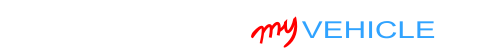## Interpreting Your Compression Test Results

It's not unusual to see some variation in the compression readings, especially on high-mileage engines.

If he variation is small enough, you're not going to notice any type of engine performance problem.

But if the variation is too big, then you're going to see a misfire problem or a rough idle problem.

The cool thing is that we can easily find out if the low compression values are causing an engine performance problem or not.

To find out, we need to figure out if the low compression values are lower than 15% of the highest compression value you got.

You can do this (figuring out the 15%) in one of two ways: You can calculate this 15% difference with pen and paper or you can use my low compression calculator. You can find the low compression calculator here: Online Low Engine Compression Calculator (at: easyautodiagnostics.com).

If you want to manually calculate the 15% difference, here's what you'll need to do:

1. STEP 1: Multiply the highest compression value by 0.15 (this is the decimal value of 15%).
2. STEP 2: Round the result to the nearest one (for example: 25.6 would become 26).
3. STEP 3: Subtract the result (the number that was rounded) from the highest compression value.
4. ANSWER: The result of this subtraction is the lowest possible compression value any cylinder can have.

Now, let me give you a more specific example: Let's say that I got the following compression readings:

Cylinder Pressure
#1 165 PSI
#2   95 PSI
#3 155 PSI
#4 175 PSI

My next step is to do the following calculation:

1. STEP 1:  175 x 0.15 = 26.25.
2. STEP 2:  26.25 = 26 (rounded to nearest one).
3. STEP 3:  175 - 26 = 149.
4. ANSWER:  149 PSI. Any cylinder with this compression (or lower) value will misfire.

Since cylinder #2 is only producing 95 PSI, I can now conclude that it's 'dead' and causing a misfire.

To find out if the lowest compression value you got from your engine compression test is within a good range, you'll need to do the same calculation. Of course, you'll need to use the highest compression value you got and not the one in the example.

Once you've found the 'dead' cylinder, the next step is to find out what's causing the low compression value. For this step, go to: TEST 2: ‘Wet’ Engine Compression Test.

## TEST 2: ‘Wet’ Engine Compression TestA low or 0 psi compression value is usually the result of one of two things:

1. The affected cylinder's piston rings are worn or damaged.
2. The affected cylinder's intake or exhaust valves are severely worn or damaged.

To find out which is the case, we don't need to take the engine apart (just yet). We can do a 'wet' engine compression test to find out.

A wet compression test simply involves adding a small amount of engine oil to the cylinder with low or 0 PSI compression and then retesting its compression.

If the compression value shoots up, then we can conclude that the low or 0 PSI compression problem is due to the cylinder having worn/damaged piston rings.

If the compression value does not increase, then we can conclude that the low compression problem is due to the cylinder having worn/damaged intake or exhaust valves.

OK, this is what you need to do:

1. 1

Add a small amount of engine oil to the cylinder that reported low compression or no compression in the ‘Dry’ compression test.

You don't have to add a lot of oil. The amount should be about 1 to 2 tablespoons of oil.

2. 2

Install the compression tester onto the cylinder.

Do not use any type of tool to tightened the compression tester. Hand tight is fine.

3. 3

When all is set up, have your helper crank the engine.

4. 4

You'll get one of two results:

1.) The compression value will go up (from the one you recorded before).

2.) The compression value will stay the same.

Let's take a look at what your test results mean:

CASE 1: The compression value shot up. This tells you that the piston compression rings are worn out and thus the problem is in the bottom end (block) of the engine in your 1.5L Mazda Protege.

CASE 2: The compression value stayed the same. This confirms that the low compression problem of the affected cylinder is due to worn or damaged cylinder head valves.

## More 1.5L Mazda Protege Tutorials

You can find a complete list of 1.5L Mazda Protege tutorials in this index:

Here's a small sample of the tutorials you'll find in the index:If this info saved the day, buy me a beer!Mazda Vehicles:

• Protege 1.5L
• 1995
1996
1997
1998